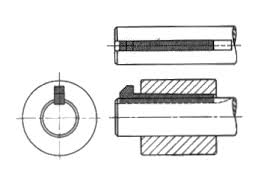# Need help calculating Shear on a 5/8 square key

vwmeche94
Need help calculating Shear on a 5/8" square key

PF,

I am trying to figure out how to calculate the shear on a 5/8" x 5/8" x 3-1/2"L standard shaft key, when the OD of the shaft it fits into is 3". A tire of ~40" dia rides on the shaft and at any given time, let's say a load of 20,000lbs is applied to put a bending moment on the shaft at the surface of the tire. If I assume the shaft is fixed for brief moment in time,
what is the maximum shear I can apply to the key before it gives (breaks)?edgepflow

A simple approach is as follows:

For the shaft to be "fixed for a brief moment" the net torque must equal zero:

So torque = F X Distance = F_tire X radius_tire = F_shaft X radius_shaft

Solve for F_shaft.

Now F_shaft will be applied in shear to your shaft key. The area in shear is : A_shear = 5/8" X 3.5 ".

And the shear stress is now:

shear_stress = F_shaft / A_shear.

When shear_stress is greater then the tensile shear stress of the shaft key, it will break (in principle).

For design, it is better to limit the calculated shear stress to about 40% of the yield stress.